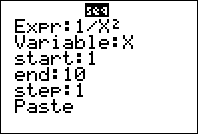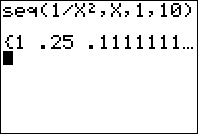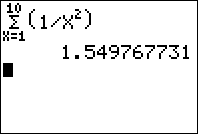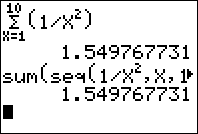Sums and Sequences on the TI-83 Plus and TI-84 Plus

Filed under Calculus, Difficulty: Medium, TI-83 Plus, TI-84 Plus.

While they may not have the calculus capabilities of the TI-89, the TI-83 Plus and TI-84 Plus have two great functions for dealing with series and sums, the “seq” and “sum” functions. This tutorial explains how to use these features effectively, as well as how to use the summation feature on newer calculators.

Sequences – The seq function

A sequence of numbers is just an ordered list of numbers, for example, {1, 2, 3, 4, 5} or {1, 2, 5, 3, 11, 2, 3}. The “ordered” part simply means that each term in the sequence is assigned a specific index. In the sequence {1, 1/2, 1/3, 1/4, …}, the second term is 1/2 and the fourth term is 1/4. Sequences can be any length, or even be infinite in length.

Sequences are handled on the TI-83 and TI-84 using the seq function. To reach this function from the home screen, press 2nd . On newer calculators, a screen will show up which will guide you through entering the function, though in any case the syntax is the same.First, enter the expression you want, next the variable you’re using, and finally the starting and ending values of your variable. The syntax will be pasted onto your screen, so press to evaluate. Note that on some older models, you might have to put quotation marks around the equation and variable name.A list containing your sequence will be returned.

A summation is a sum of numbers that are typically defined by a function. For example, saying “the sum from 1 to 4 of n²” would mean 1²+2²+3²+4² = 1 + 4 + 9 + 16 = 30. Sums can also be infinite, provided that the terms eventually get close enough to zero–this is an important topic in calculus. For example, adding up the sum of 1/n does not converge, whereas the sum of 1/n² converges to π²/6, a rather unexpected result.

There are a couple of ways to evaluate sums on your calculator. Calculators with newer operating systems actually have a summation function, which can by reached by pressing . With it you can use your arrow keys to select your counting variable (typically X), set the limits, and enter the expression you want to evaluate.If your calculator doesn’t have this feature, you can perform the same operation by summing up a sequence: Press 2nd to reach the List MATH menu, to select sum, then select the seq function as above. Press to evaluate.Unfortunately, The TI-83 Plus and TI-84 Plus don’t have a method for evaluating infinity sums, but if you evaluate a convergent summation to enough terms (like 100), it should approximate the infinite sum pretty well. For example. the sum from X=1 to 100 of 1/x² is 1.635, and the infinite sum is around 1.645.

2 Responses to Sums and Sequences on the TI-83 Plus and TI-84 Plus

1.mafy

very useful, thanks

•John (Nate) Whitten

Thanks. I had jacked around with the calculator for a while, knowing that I could sum a finite series or a quickly convergent infinite series. I could not remember. I typed in the question and had the answer in 2 shakes of a sheep’s tail. thanks. nw